# We Helped With This Python Programming Assignment: Have A Similar One?

SOLVEDCategory Programming Python Graduate Solved Python Assignment

## Short Assignment Requirements

i need to solve the laplace equation using th jacobi and gauss-seidel schemeproblem 1.Solve this problem for N=11, 21, 41, and 101. Plot the error versus the iteration count on a semi log plot. Do this for theJacobi and Gauss-Seidel schemes.ðœ™{ð‘–,ð‘—} = 0.25 ( ðœ™ð‘–+1,ð‘— + ðœ™ð‘–âˆ’1,ð‘— + ðœ™ð‘–,ð‘—+1 + ðœ™ð‘–,ð‘—âˆ’1)see the attached file for more details

## Assignment Description

Assignment 2: Laplace Equation

### DESHAM MANIDEEP REDDY

163010004

Problem definition:

In mathematics, Laplace's equation is a second-order partial differential equation named after Pierre-Simon Laplace who first studied its properties. This is often written as: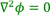Where the operator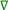I Cartesian coordinate system can be written as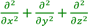therefore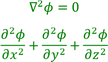Here in our problem, we are concerned with 2 D version of Laplace equation which is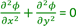Although there are many analytical techniques, we use numerical techniques to solve the above problem. Let’s discretize the above equation with central difference scheme. We get,Similarly,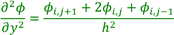Where i, j are indices along X and Y axes respectively. Substituting the discretized formulas in the above Laplace equation we get,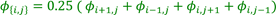### Problem: 1

Solve this problem for N=11, 21, 41, and 101.  Plot the error versus the iteration count on a semi log plot.  Do this for the Jacobi and Gauss-Seidel schemes.

#### Jacobi scheme

Jacobi scheme is an iterative scheme where we take an initial guess of solution (normally zeros) and successively improvise the values after every iteration. Here we use the values from previous iteration and move forward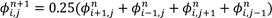The error in an iteration step is found as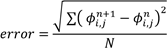The iterations are continued until the error is less than the value 2*(machine epsilon) the value of which in our case is 2 ∗ 10−16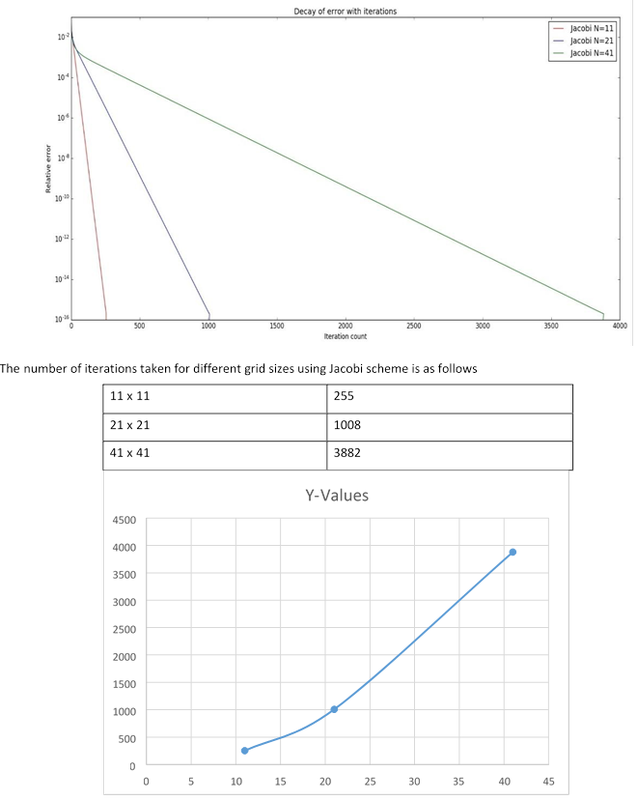#### Gauss Seidel

Gauss seidel scheme is similar to the Jacobi scheme, the only difference is that here we are using the latest information as and when it is available.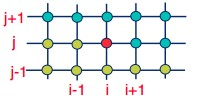From the above figure, we may observe that as we are going to find the value of 𝜙 at i, j the values at i-1,j and I,j-1 are known already. Jacobi method does not use this updated information. Here in Gauss-siedal method using this updated information leads to faster convergence.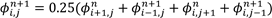### Problem: 2

For the SOR scheme, choose N=41.  Fix the number of iterations to 20 and hunt for a suitable w value between 0 to 2 in steps of 0.1.

#### Successive Over Relaxation (SOR)

Successive over relaxation technique uses the concept of accelerating convergence. The above two methods namely Jacobi and Gauss-siedal method takes lot of CPU time to converge if the size of grid becomes larger and larger. SOR is an acceleration technique that works by taking by taking a linear combination of the new iterate and old iterate.

In this method we take a new variable called over-relaxation parameter  ‘𝜔’ . Where 𝜔 (0,2) . Our new algorithm is a two step process

𝜙𝑖∗,𝑗 = 0.25(𝜙𝑖𝑛+1,𝑗 + 𝜙𝑖𝑛−+11,𝑗 + 𝜙𝑖𝑛,𝑗+1 + 𝜙𝑖𝑛,𝑗+−11)

𝜙𝑖𝑛,𝑗+1 = 𝜔 𝜙𝑖∗,𝑗 + (1 − 𝜔)𝜔 𝜙𝑖∗,𝑗

Case:1 choosing N=41, Number of iterations = 20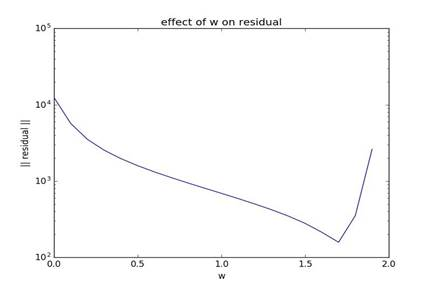Hunt for a suitable w value between 0 to 2 in steps of 0.1 resulted in

𝜔𝑜𝑝𝑡𝑖𝑚𝑢𝑚=1.6

### Problem: 3

Repeat problem 2 with the number of iterations set to 50 and 100.  Check if the plot changes.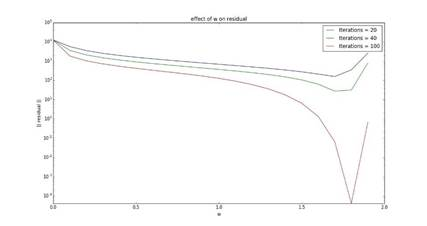From the above we may observe that the plot in fact changes as the number of iterations changes. Below table shows the

variation of 𝜔𝑜𝑝𝑡𝑖𝑚𝑢𝑚

 Number of iterations 𝜔𝑜𝑝𝑡𝑖𝑚𝑢𝑚 20 1.7 40 1.7 100 1.8

### Problem: 4

Once an approximate w_{opt} is found, hunt for a better estimate for the optimal in the range, (w_opt - 0.1, w_opt + 0.1) with steps of w in 0.01.  Use 50 iterations.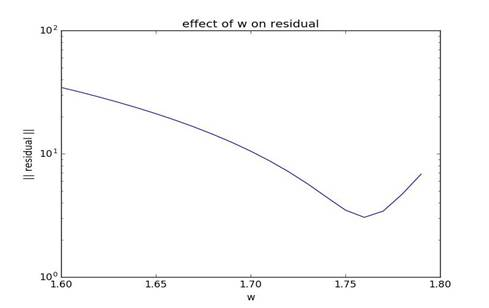The grid size taken is 41 x 41 and the number of iterations are 50.

The value of  𝜔𝑜𝑝𝑡𝑖𝑚𝑢𝑚 is found to be 1.75

### Problem: 5

Does the w_opt change if N=101 and with a total of 100 iterations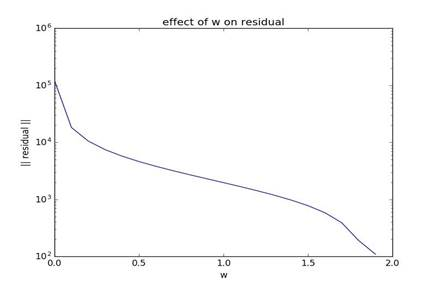From the adjacent figure, we may observe that for N=50 (100 is not working in my computer) and number of iterations =100.

We may observe that 𝜔𝑜𝑝𝑡𝑖𝑚𝑢𝑚 in fact changes.

### Problem: 6

Repeat the hunt for an optimal w_opt (with w = linspace(1, 2, 11)) but this time calculate the number of iterations it takes to converge to machine epsilon instead of the error.  Plot the number of iterations vs. w.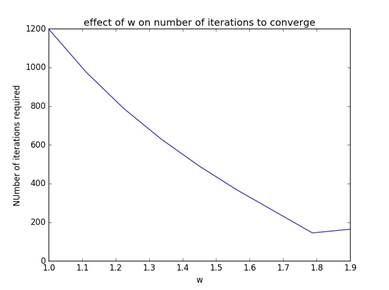The adjacent figure shows the number of iterations required for a particular 𝜔 value so that the error between two successive iteration steps is less than 2 ∗ 10−8  .

The problem statement to obtain the grid is as follows:

Grid size = 50 x 50

𝜔 = linspace(1,1.9,9)

Error condition: error < 2∗ 10−8

The value of 𝜔 for which minimum number of iterations are taken to converge is 𝜔 = 1.78.

### Problem: 7

Having found the optimal w, take N=101 and solve this using all the three schemes and plot the error versus the number of iterations in a semi-log plot.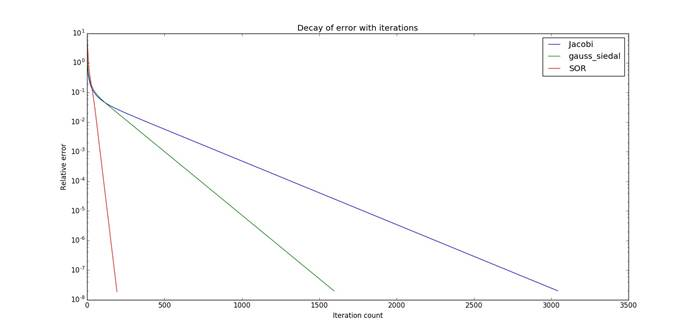The above plot shows the different number iterations required by different methods to arrive at a particular error condition. The number of iterations required by different methods are as follows:

 Method Number of iterations required Jacobi method 3042 Gauss siedal 1600 SOR 192

From the above table we may observe that Jacobi method takes 15 times the iterations required by SOR technique.

Is it free to get my assignment evaluated?

Yes. No hidden fees. You pay for the solution only, and all the explanations about how to run it are included in the price. It takes up to 24 hours to get a quote from an expert. In some cases, we can help you faster if an expert is available, but you should always order in advance to avoid the risks. You can place a new order here.

How much does it cost?

The cost depends on many factors: how far away the deadline is, how hard/big the task is, if it is code only or a report, etc. We try to give rough estimates here, but it is just for orientation (in USD):

 Regular homework \$20 - \$150 Advanced homework \$100 - \$300 Group project or a report \$200 - \$500 Mid-term or final project \$200 - \$800 Live exam help \$100 - \$300 Full thesis \$1000 - \$3000

How do I pay?

Credit card or PayPal. You don't need to create/have a Payal account in order to pay by a credit card. Paypal offers you "buyer's protection" in case of any issues.

Why do I need to pay in advance?

We have no way to request money after we send you the solution. PayPal works as a middleman, which protects you in case of any disputes, so you should feel safe paying using PayPal.

Do you do essays?

No, unless it is a data analysis essay or report. This is because essays are very personal and it is easy to see when they are written by another person. This is not the case with math and programming.

Why there are no discounts?

It is because we don't want to lie - in such services no discount can be set in advance because we set the price knowing that there is a discount. For example, if we wanted to ask for \$100, we could tell that the price is \$200 and because you are special, we can do a 50% discount. It is the way all scam websites operate. We set honest prices instead, so there is no need for fake discounts.

Do you do live tutoring?

No, it is simply not how we operate. How often do you meet a great programmer who is also a great speaker? Rarely. It is why we encourage our experts to write down explanations instead of having a live call. It is often enough to get you started - analyzing and running the solutions is a big part of learning.

What happens if I am not satisfied with the solution?

Another expert will review the task, and if your claim is reasonable - we refund the payment and often block the freelancer from our platform. Because we are so harsh with our experts - the ones working with us are very trustworthy to deliver high-quality assignment solutions on time.

## Popular Solved Assignments Like This

Customer Feedback

"Thanks for explanations after the assignment was already completed... Emily is such a nice tutor! "

Order #13073